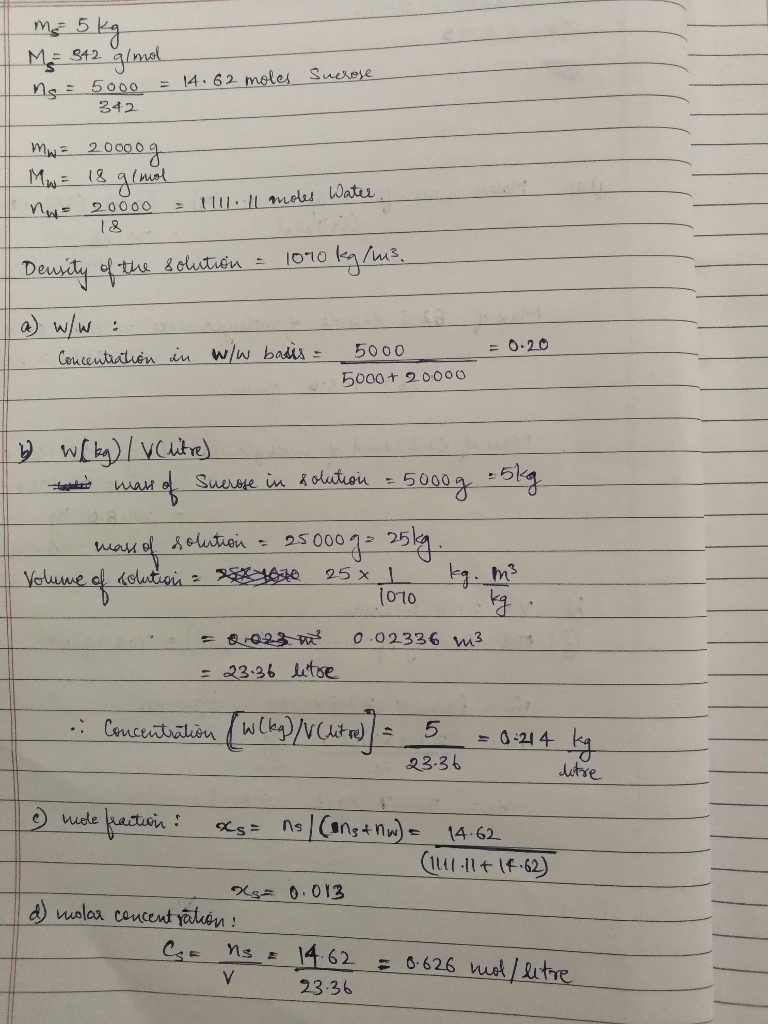# An amount of 5 kg of sucrose (MW = 342 g/mol) is dissolved in 20,000 g of water. The density of the sucrose solution is 1070 kg/m3 . Estimate the sucrose concentration in the solution in (a) w/w, (b) w (kg)/V (L), (c) mole fraction, and (d) molar concentration.

Question-AnswerCategory: Material And Energy BalanceAn amount of 5 kg of sucrose (MW = 342 g/mol) is dissolved in 20,000 g of water. The density of the sucrose solution is 1070 kg/m3 . Estimate the sucrose concentration in the solution in (a) w/w, (b) w (kg)/V (L), (c) mole fraction, and (d) molar concentration.

An amount of 5 kg of sucrose (MW = 342 g/mol) is dissolved in 20,000 g of water. The
density of the sucrose solution is 1070 kg/m3
. Estimate the sucrose concentration in the
solution in (a) w/w, (b) w (kg)/V (L), (c) mole fraction, and (d) molar concentration.